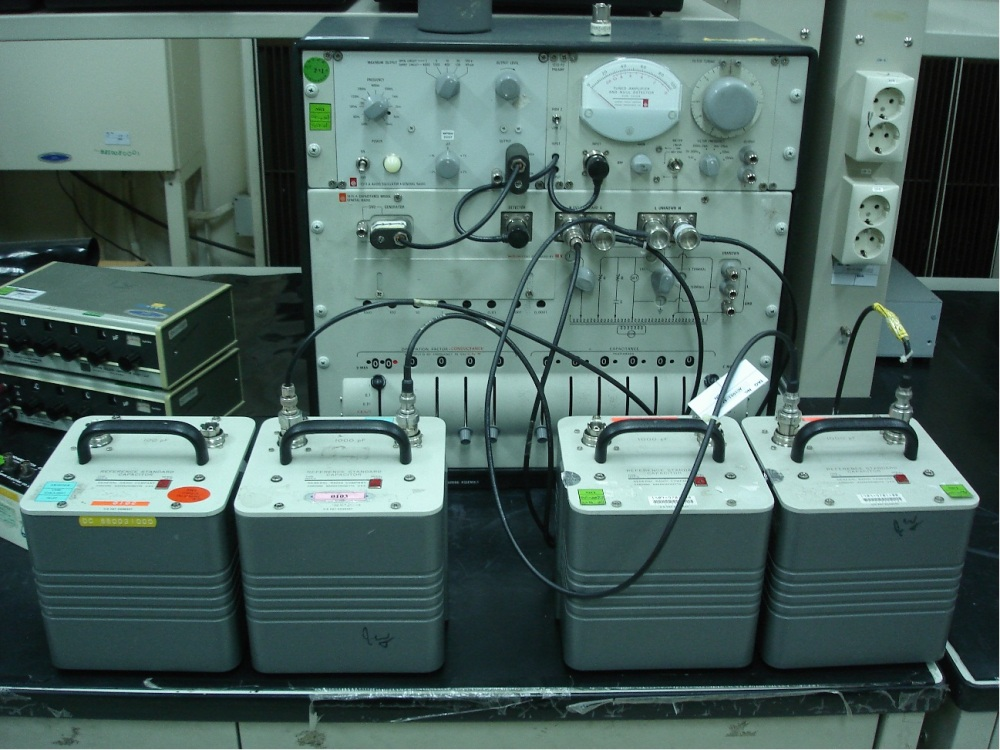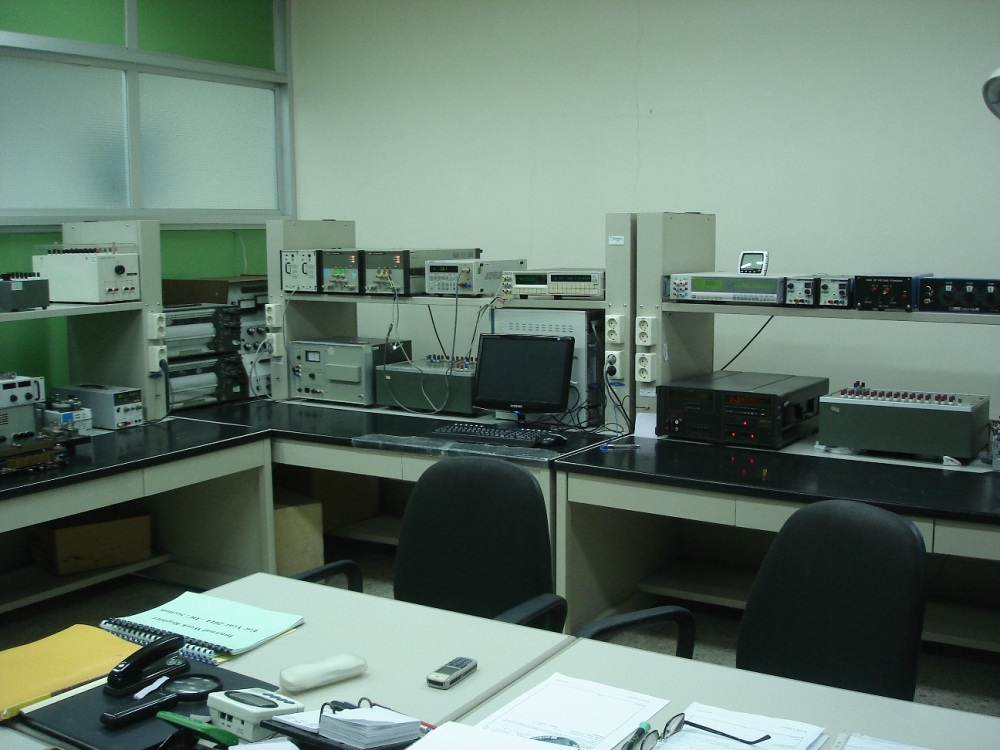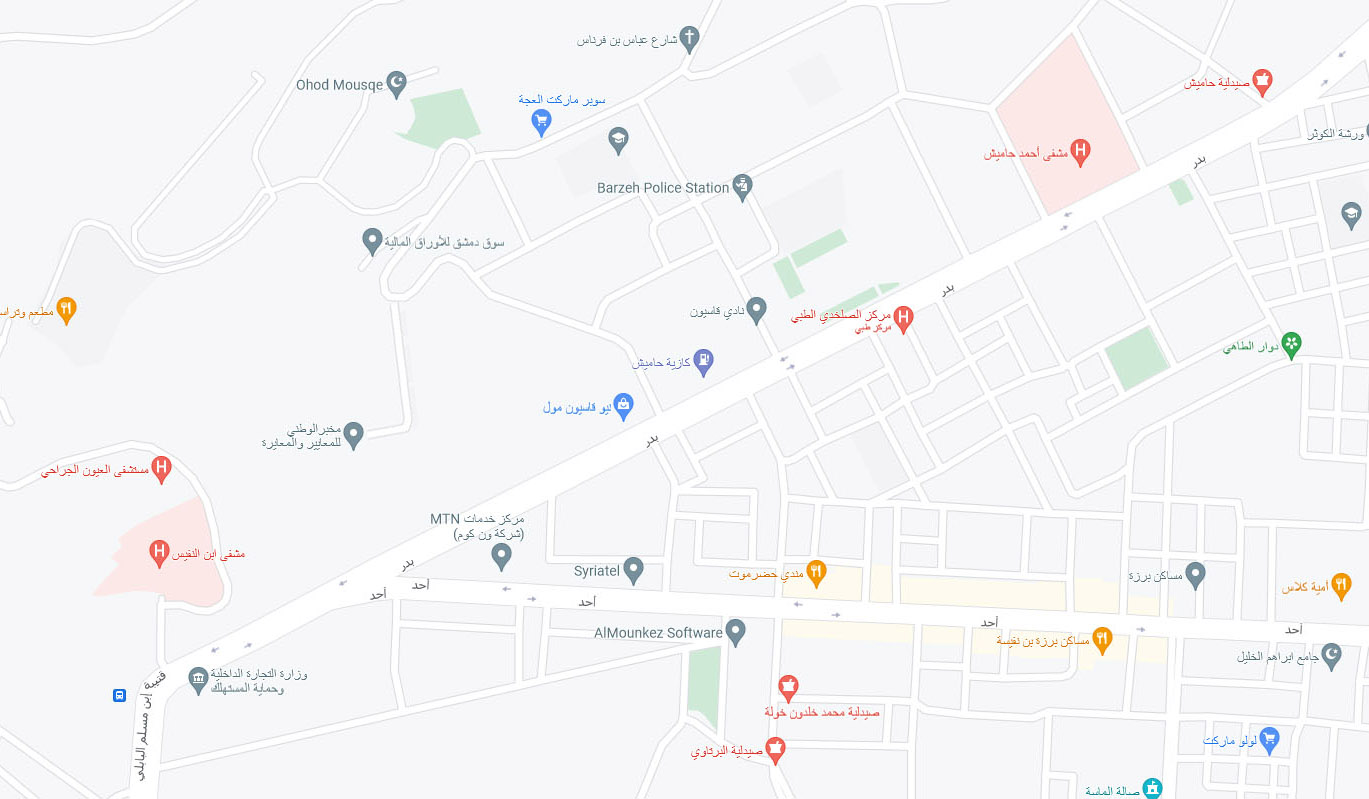# Direct Current (DC) MeasurementsNSCL maintains the following standards as the reference measurement standards of DC voltage, DC current, resistance and capacitance for Syria and for the dissemination of the related units:

• Two Fluke 732A as the reference measurement standards of DC voltage. The back to back method, using a digital voltmeter, is used to perform measurements.
• A current source serially connected to a set of Yokogawa 2792 resistors as the reference measurement standard of DC current, where a reference digital multimeter (Fluke 8508A) is used to measure the dropped voltage.
• A set of five double-wall standard resistors Yokogawa 2781 as the reference measurement standard of resistance, where a current comparator resistance bridge (Guildline 9975) is used to perform measurements.
• A set of four-dry nitrogen capacitors GR 1404A and GR 1404B as the reference measurement standard of capacitance, where a capacitance measuring assembly bridge (GR 1620-AP) is used to perform measurements.
 Measured quantity/Calibrated item Range Expanded uncertainty (K=2) DC voltage sources Standard  cells 1.018 V 1.9 ppm Solid state voltage standards 1 V, 1.018 V, 10 V (1.3 to 2.1) ppm DC voltage calibrators 1 mV to 1000 V (2.4 to 830) ppm DC voltage meters Standard DC voltage meters 100 mV to 1000 V (2.8 to 17) ppm DC voltage meters 1 mV to 100 mV (17 to 830) ppm DC current Sources 100 μA to 100 A (16 to 300) ppm Measuring instruments 100 μA to 100 A (14 to 300) ppm DC resistance Fixed standard resistors and resistance boxes 10 mΩ to 10 MΩ (8 to 30) ppm Current shunts 1 mΩ to 10 mΩ (22 to 300) ppm Capacitance Fixed capacitors and capacitance boxes 100 pF to 10 μF at Freq 1 kHz (20 to 380) ppm Capacitance bridges & capacitance meters 1 pF to 10 μF at Freq 1 kHz (110 to 380) ppm Dissipation factors > 1 ∙ 10-6 to 1 at Freq 1 kHz 1000 ppm . tan δ + 50 ppm

How To Request Calibration Services

## Finding Us

• National Standards and Calibration Laboratory
• Masaken Barzeh
Pre-fabricated house area
Damascus - Syria
•